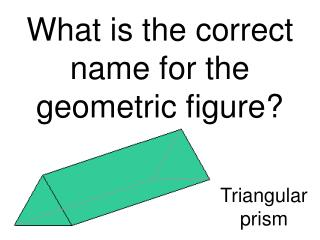DownloadDownload PresentationWhat is the correct name for the geometric figure?

# What is the correct name for the geometric figure?

Télécharger la présentation## What is the correct name for the geometric figure?

- - - - - - - - - - - - - - - - - - - - - - - - - - - E N D - - - - - - - - - - - - - - - - - - - - - - - - - - -
##### Presentation Transcript

1. What is the correct name for the geometric figure? Triangular prism

2. What is the correct name for the geometric figure? Pentagonal prism

3. What is the correct name for the geometric figure? Rectangular solid

4. What is the correct name for the geometric figure? Triangular prism

5. Which figure has five lateral faces that are triangles?A. Pentagonal prismB. Pentagonal pyramidC. Rectangular prismD. Rectangular pyramid

6. How are cones and cylinders alike?A. They both have circular baseB. They both have two bases.C. They both have a vertex at the top.D. Their bases are both parallel to each other.

7. When comparing the volume of a prism and a pyramid with the same base area and height, which is true? A. The V of the pyramid is 1/3 the volume of the prism.B. The V of the pyramid is ½ the volume of the prism.C. The V of the pyramid is 2 times the V of the prism.D. The V of the pyramid is 3 times the V of the prism.

8. Which is true about parallelograms?A. All s have 4  sides.B. All s have 4 right s.C. All s have only 1 pair of opp sides parallel.D. All s have pairs of opposite sides that are both ║ and .

9. If two figures are similar, which is true?A. Corresponding s are .B. Corresponding sides are .C. All corresponding s of the figures are proportional and all corresponding sides are .D. All corresponding s of the figures are  and all corresponding sides are proportional.